## Force in a Magnetic Field:

Force in a Magnetic Field – If a conductor of length l metres carrying current I amperes is placed in a magnetic field of intensity B (tesla), and if B is perpendicular to I, the force acting on the conductor is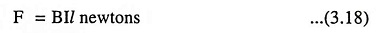If N electrons are contained in a length l of the conductor [Fig. 3.6 (a)] and if an electron takes time T seconds to travel a distance of l metres in the conductor, the total number of electrons passing through any cross section of wire in unit time is N/T.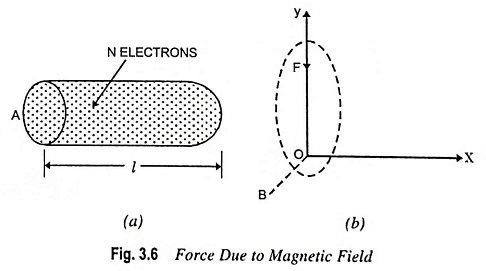Thus, the total charge per second passing any point, which, by definition, is the current in amperes, is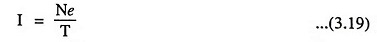Thus, the force due to Magnetic Field (Fig. 3.6(b)]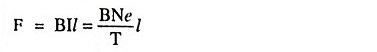Furthermore, since l/T is the average, or drift, speed v metres per second of electrons, the force experienced by each electron due to magnetic field is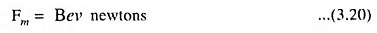The subscript m indicates that the force is of magnetic origin.

Scroll to Top